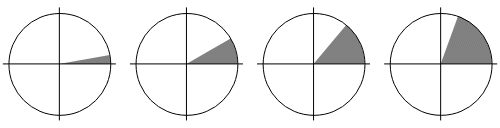Search IntMath
Close

# What is an Acute Angle in Geometry?In geometry, an acute angle is an angle that is less than 90 degrees. It is one of the few types of angles that can be found in geometric shapes. An acute angle is usually represented by the lowercase letter 'a' with a line over it, or the symbol ?. The measure of an acute angle is always less than 90 degrees, and greater than 0 degrees.

## Acute Angles and Right Angles

A right angle is an angle that measures exactly 90 degrees. It is represented by the symbol ?. Acute angles are the exact opposite of right angles. This means that an acute angle is less than 90 degrees, while a right angle is exactly 90 degrees. The two angles together make a straight line, which is 180 degrees.

## Acute Triangles

An acute triangle is a triangle that has all acute angles. This means that all three angles inside the triangle are less than 90 degrees. An acute triangle is also known as an acute-angled triangle. A right triangle is a triangle that has one right angle and two acute angles. An obtuse triangle is a triangle that has one obtuse angle and two acute angles.

## Obtuse Angles

An obtuse angle is an angle that is greater than 90 degrees and less than 180 degrees. It is represented by the symbol ?. An obtuse angle is the exact opposite of an acute angle, as an acute angle is less than 90 degrees and an obtuse angle is greater than 90 degrees. An obtuse triangle is a triangle that has one obtuse angle and two acute angles.

## Complementary Angles

Complementary angles are two angles that add up to 90 degrees. They can also be referred to as �complements�. The two angles can be either acute or obtuse angles, as long as they add up to 90 degrees. Complementary angles are an important part of geometry, as they can be used to solve problems and measure angles in shapes.

## Practice Problems

1. What is the measure of an acute angle?
Answer: An acute angle has a measure of less than 90 degrees.

2. What is an acute triangle?
Answer: An acute triangle is a triangle that has all acute angles.

3. What is the measure of a right angle?
Answer: A right angle has a measure of exactly 90 degrees.

4. What is the difference between an acute angle and an obtuse angle?
Answer: An acute angle is less than 90 degrees, while an obtuse angle is greater than 90 degrees.

5. What are complementary angles?
Answer: Complementary angles are two angles that add up to 90 degrees.

6. What is the measure of an obtuse angle?
Answer: An obtuse angle has a measure of greater than 90 degrees and less than 180 degrees.

7. What is a right triangle?
Answer: A right triangle is a triangle that has one right angle and two acute angles.

8. What is an obtuse triangle?
Answer: An obtuse triangle is a triangle that has one obtuse angle and two acute angles.

9. How can complementary angles be used?
Answer: Complementary angles can be used to solve problems and measure angles in shapes.

10. How can an acute angle be represented?
Answer: An acute angle can be represented by the lowercase letter 'a' with a line over it, or the symbol ?.

## Summary

In geometry, an acute angle is an angle that is less than 90 degrees. It is usually represented by the lowercase letter 'a' with a line over it, or the symbol ?. Acute angles are the exact opposite of right angles, which measure exactly 90 degrees. An acute triangle is a triangle that has all acute angles, while a right triangle is a triangle that has one right angle and two acute angles. An obtuse angle is an angle that is greater than 90 degrees and less than 180 degrees, and an obtuse triangle is a triangle that has one obtuse angle and two acute angles. Complementary angles are two angles that add up to 90 degrees, and can be used to solve problems and measure angles in shapes.

In this article, we discussed what an acute angle is in geometry, as well as its relationship to right angles, acute triangles, obtuse angles, and complementary angles. We also included some practice problems to help you understand the concepts better.

## FAQ

### What is an acute angle?

An acute angle is an angle less than 90�.

### What is the formula for finding the measure of an acute angle?

The formula for finding the measure of an acute angle is ? = 90� - x, where x is the measure of the angle.

## Problem SolverThis tool combines the power of mathematical computation engine that excels at solving mathematical formulas with the power of GPT large language models to parse and generate natural language. This creates math problem solver thats more accurate than ChatGPT, more flexible than a calculator, and faster answers than a human tutor. Learn More.